# A Multi-objective Hybrid Algorithm for Planning Electrical Distribution System

A Multi-objective Hybrid Algorithm for Planning Electrical Distribution System
Department of Electrical and Electronics Engineering, Anna University, Chennai 600025, India

Department of Electronics and Communication Engineering, Anna University, Chennai 600025, India

Corresponding Author Email:
rajeshkannan.mt@gmail.com
Page:
377-387
|
DOI:
https://doi.org/10.18280/ejee.224-509
15 June 2020
|
Accepted:
26 September 2020
|
Published:
30 October 2020
| Citation

OPEN ACCESS

Abstract:

In this manuscript we establish a multiple-objective gravitational search algorithm (GSA) and Tabu heuristic search to plan electrical distribution system. GSA is minimized the Distribution Generator (DG) investment cost, cost of distribution system loss, operation as well as DG maintenance cost with help of appropriate constraints. So the optimum sizing of DG is planned in the optimum location with reduced cost. Here, the Gravitational Search Algorithm (GSA) accelerates the speed of convergence to combination of Tabu heuristic search method search strategy. As established multipurpose hybrid algorithm to plan electrical distribution system performed at MATLAB / simulink platform as well as efficiency evaluated to compare it other methods. The outcomes of comparison determine the advantage of established method as well as confirm its potential to resolve issue.

Keywords:

GSA, Tabu search, DG, operation and maintenance cost, investment cost

1. Introduction

Onset of an aggressive electricity market coupled with hi-tech growth and ecological contaminations has triggered the progress of DG, especially the renewable energy resources . No wonder, the electric power distribution system design has emerged as significant technology to power utility [2, 3]. As main focus of the current power system economics is targeted at ensuring a distribution system with the least installation and process outlay . The challenges facing the distribution system planning comprise various factors such as taking an appropriate decision on optimal numbers as well as localities supply substations as well as finest method of linking load nodes with this substation by means of inter-linking of feeders . This calls for a proper planning which will, in the long run, usher in considerable reduction in costs without in any way compromising customer delight. Consequently, distribution system plan consists of virtually turn a multi-objective optimization issue [6, 7]. Conversely, one of the vital issues faced by distribution system planning models is that the number of optimal plans with least expenditure is limited to one only .

The central aim of the distribution system plan (DSP) issue is targeted at furnishing a dependable as well as cost effectual service with clients simultaneously guaranteeing voltages as well as power quality maintained at the benchmark levels [9-11]. The cost reduction encompasses several factors including capital and overheads of recently established and the existing facilities, expenditure involved by the purchase of power from other electric enterprises, and the amount spent on account of electric system power losses . The distribution power system planning technique involves two significant phases. The former phase is the preliminary system planning and the latter is the optimization planning for consistency . The smart grid incorporation of dispatch able renewable units DG like biomass generators has become as remarkable alternatives to satisfy ever zooming load demands, at the same time radically perform customer reliability as well as decreasing entire releases .

Anyhow the reactive power planning for a distribution network is effortlessly carried out with the proper positioning of shunt capacitor banks . The evolutionary calculation methods are employed as solution techniques in a lion’s share of the Pareto-based multi-objective planning schemes fascinated by their multi-point exploration potency, facilitating the achievement of place of non-dominated solutions [16-18]. Central targets of multi-objective algorithms are focused on inching closer to the place of optimal solutions that would lead with place of diversified solutions. Hence it can be stated that the multi-objective algorithm sets its bar with the lofty motive of an instantaneous achievement of sterling convergence [19, 20].

The manuscript establishes a multiple-objective gravitational search algorithm as well as taboo heuristic search to plan electrical distribution system. GSA [21-23] is minimized as Distribution Generator (DG) investment cost; distribution system cost loss, operation as well as DG maintenance cost with help of appropriate constraints. So the optimum sizing of DG is planned in the optimum location with reduced cost. Here, the Gravitational Search Algorithm (GSA) accelerates the speed of convergence to combination of Tabu heuristic search method search strategy. The specified explanation of established hybrid method as well as UPFC power flow model is discussed at section 3. Previously, current investigation manuscript is introduced at section 2. An outcome as well as conversation is suggested at section 4. Section 5 concludes the manuscript.

2. Recent Research Works: A Brief Review

Literature is flooded with innumerable investigations of relevance based on Distribution System planning with several objective types, a few of which are discussed below. Jose L. Rueda et al.  have judiciously brought out an all-inclusive technique to estimate an optimum transmission system expansion plan taking due account of the enrichment of small-signal solidity by means of wide-ranging use of accessible as well as designed transmission system property. Vibrant model of transmission network operation plan (TNOP) was resolved in accordance with a blend of the Mean-Variance Mapping Optimization (MVMO), as well as standard dynamic scheduling technique entrenched to heuristic process. In addition, probabilistic Eigen analysis depend on recursive approach was launched with estimate as optimal control techniques were significantly applicable with augmentation of system small-signal solidity excellence all through the planning perspective. Numerical outcomes recount the success stories of the perspective and the efficiency of the innovative technique in ushering in optimal strategies of least expenditure simultaneously tackling successfully the volatility risk linked to badly damp minimal frequency electromechanical oscillations.

With inherent genius, Ganguly et al.  have carved an innovative vibrant programming technique to multi-objective plan of electrical distribution systems. In proposed design envisions the estimation of optimal feeder route as well as branch conductor dimensions of distribution system to concurrent optimization of expenditure as well as consistency. Multiple planning targets covered reduction of various facets encompassing establishment and running expenses along with reduction in overheads due to delay. The former objective task embraced several areas such as the establishment expenses of novel feed branches as well as substations, preservation overheads of current as well as novel feed branches, and payment towards loss of energy. On contrary, the latter objective task estimates consistency of distribution network according to related expenditure due to delay for the entire branches including which included the expenditure towards non-delivered energy, maintenance cost, as well as customer loss cost on account of delays. Thus, dynamic scheduling depends on planning algorithm to optimize of routes feed as well as size of branch conductor was launched.

Naderi et al.  have effectively shaped a novel technique to consider DGs at DSP issue. The innovative model, optimal power flow (OPF) was envisioned as reduce capital expenditure to system renovation, running as well as preservation expenses, as well as payments towards damages in handling the load expansion for the design domain. The idea encapsulated in the term “dynamic” pointed to the planning for a definite interval which was envisaged in the innovative dynamic distribution system planning. Moreover, a customized genetic algorithm was also employed to hit upon the optimal topology solution.

Samui et al.  have significantly ushered in an analysis at function of consistency deliberation at distribution system design as well as plan expenditure of radial distribution system. Direct investigation method was used for optimum planning envisaging only the significant reduction of planning outlay. Moreover, the idea of principle of optimality thesis enables the direct technique become highly effective in computation by bringing down the whole numbers of radial path. Reliability index were determined to assess consistency of systems to diverse feed structures. Innovative technique was also experimented to optimal feed route by duly altering the substations number that furnished data on swapping among optimality as well as dependability of system structure.

Cossi et al.  have competently offered the paradox of planning primary distribution networks as multi-purpose mixed-integer nonlinear programming model (MINLP). The purpose tasks of the proposed method were the investment expenses in the development and processes of main distribution networks, in addition to consistency costs of what happened in unforeseen. Consistency costs were estimated by calculate the energy not supplied in view of maintenance as well as switching processes at distribution network carried out to segregate and reassign loads at segment affected to eternal errors. In order to address the growth concerns of main network, a multi-objective reactive taboo search algorithm (MO-RTS) was envisioned. Domain theories were applied to gather the Pareto optimal boundary at MO-RTS. Issue of positioning disconnecting switches to restore distribution system and reduce the waste of energy not supplied during the incidence of eternal errors in the system was addressed simultaneously with the problem of planning the growth of the network with the help of a genetic algorithm committed.

da Rocha et al. proposed a dynamic multi-year model to Transmission Expansion Planning, TEP, a complexity to recognize the appropriate set of projects and its programming all along the planning horizon . As fitness activity includes the operation as well as investment rate and penalty term set, a candidate's plans were determined. These provisions were correlated to loss level, non-zero values to power not provided, that is, to all system as n - 1 unexpected event, financial restrictions, plus the number of projects to execute at every year or in the whole horizon and potential to hold not only the predictable demand, yet precariousness that disrupts the required predictions. To carry out distinct problem, an advanced algorithm is introduced namely PSO algorithm. This algorithm comprises a developmental embracing of the PSO regulation and further several corrections to assure that participative progression of each candidate key is technically possible given its distinct nature.

In a framework of multiple objective optimization, issue of Multistage Distribution Network Expansion Planning (MDEP) at being there of Distributed Generations (DG), is presented by Gitizadeh et al.  These patterns combine two goals. To reduce investment costs as well as operating costs, and maximize the reliability index. They also projected an updated model subject with AC power flow restrictions to acquire best feeder configuration (add and remove lines) that contains best derived lead size, alternative lead for backup feeders, as well as created DG power. To determine the Energy-Not-Supplied index (ENS), systematic method based on graph theory was performed as an extra objective for the proposed MDEP reliability problem. Hybrid Particle Swarm Optimization (PSO) and Shuffled Frog Leaping (SFL) algorithm was run to recognize optimal Pareto solutions to issue of multiple MDEP targets.

Duque et al.  have illustrated abio-inspired metaheuristic known as monkey search (MS) to the planning of electrical energy distribution systems (EEDS). The algorithm applied for the planning of energy distribution systems, called modified monkey search (MMS), was an improvement of the original MS method with the purpose of a better fit to the EEDS applications. The planning problems covered by the MMS application are the optimal allocation of fixed and switched capacitor banks, as well as diverse kind of meters as Phasor measurement units and smart meters to aid the system state estimation process.

Xie et al.  have explained a new expansion planning model for an active distribution system considering multiple active network management schemes, where demand management considering the optimal load-shedding direction was introduced into the planning model. This model optimizes the investment decisions of all the infrastructures as well as the corresponding operational strategies in a coordinated manner. Therefore, the final solution was identified by the optimal type, location and size of the feeders, distributed generators, capacitor banks, static VAR compensators, voltage regulators, energy storage systems, and transformer substations. To incorporate the optimal load reduction into active network management, the optimal load-shedding direction was introduced, and an iterative method is developed to compute it.

Gantayet et al.  have established the placing and sizing of distributed energy resources (DERs) have a significant impact on system behavior. For the presented DG allocation problem, a multiobjective function was created by fusing in two single objective functions of loss reduction and voltage stability development. Genetic algorithm (GA) was employed to solve the multiobjective optimization problem.

Normally, the distribution system plan model devises to objective function. The objective function varies such as minimizing the cost of installation, energy loss cost, and maximization of profit. One of the significant characteristics at competitive power market is examined as other objectives for stability of distribution system. Stability of distribution system is formed to total cost of service interruption, customer interruption, as well as contingency load loss rate. Different method is employed to optimize as objective cost function as well as stability of planning model. That approaches have been solved by single solution problem or multi-objective decision making problem. In this planning model, the main challenge formulates a solution method since objective function is normally non-linear, non-convex, and not differentiable by separate and continuous resulting variables. The complexity increases by means of advanced proportions that depend on number of bus at system. Complexity is overcome by two ways of solution strategies that are deterministic and optimization algorithms. Deterministic algorithm depends on mathematical optimization procedure which may generate similar output to certain input for all time. Hence, it would solve the problem by only nonlinear, dynamic, and mixed type programming methods. But, the optimization algorithm is able to generate a feasible solution with issue at various practical situations, yet solution is varied by randomly. The optimization algorithms are like genetic algorithm, Tabu search, artificial immune system, particle swarm optimization, honey bee mating optimization and etc. But, these algorithms have the convergence problem which is overcome by introduced new search techniques. The above optimization techniques are used as solution strategies in most of the Pareto-based multi-objective planning approaches due to their multi-point search capability, which helps to obtain a set of non-dominated solutions in a single run. However, the major drawback of an optimization technique is that the convergence is not always guaranteed. On the contrary, the deterministic algorithms are well known for their good convergence characteristics. Moreover, it is reported in the above literature works that the computation time of optimization techniques is reasonably higher than that of dynamic programming for the same distribution system planning problem.

The key contributions of this paper are:

• Multiple-objective gravitational search algorithm (GSA) and Tabu heuristic search to plan electrical distribution system.
• GSA is minimized the Distribution Generator (DG) investment cost, cost of distribution system loss, operation as well as DG maintenance cost with help of appropriate constraints. So the optimum sizing of DG is planned in the optimum location with reduced cost.
• Here, the Gravitational Search Algorithm (GSA) accelerates the speed of convergence to combination of Tabu heuristic search method search strategy.

The proposed multi-purpose function is briefly explained at next section 3.

3. Formulation of Multi-Objective Function

These sections describe the objective of our established operation, which formulated by the multi-objective function. Objective function varies as reduction of DG installation cost, energy loss cost as well as maximization of profit. One of significant characteristics at competitive energy market is examined as other objectives for stability of distribution system. Stability of the distribution system is made up of the total cost of service interruption, customer interruption as well as contingency load loss rate. Different method is employed to optimize objective cost function as well as stability of planning model. Those approaches have been solved using either a single solution problem or a multi-objective decision making problem. In this planning model, the main challenge is to formulate a solution method since objective function is usually nonlinear, non-convex, and not differentiable by separate and continuous resulting variables. Here, the multi-objective model is developed to minimize cost of DG installation, the cost losses, operation cost as well as maintenance. Needed multi-objective function is described at equation below (1).

Fitness$=\Psi=\operatorname{Min}\left\{f_{1}, f_{2}, f_{3}\right\}$      (1)

here $f_{1}=C_{D G}^{i n v}$  denotes DG investment cost; $f_{2}=C_{D G}^{o \& m}$ denotes operating and maintenance cost of DG and $f_{3}=C_{L}$ denotes cost of losses; the description about the proposed multi-objective function is given  in following Eqns. (2), (3) and (4). The DG investment cost calculation is needed to perform 8760hour analysis.

$C_{D G}^{i n v}=\sum_{i=1}^{N_{b} \Sigma} C_{i}^{i n v}\left\{\frac{P_{D G i}^{\max }}{C_{\text {annual}} 8760}\{\}\right\}$    (2)

With, $C_{\text {annual}}=\left[\frac{(1+r)^{T}-1}{r(1+r)^{T}}\right]$ is the annualized factor; $N_{b}$ is the whole number of bus possible with associate DG; $P_{D G i}^{\max }$  is total DG units capacity limit at bus $i(M V A)$ ; $C_{i}^{i n v}$ denotes DG investment cost $(\$ / M V A)$; r denotes discount rate as well as$T$denotes horizon planning period (year). The operating and maintenance cost equation is described by the following.$C_{D G}^{o \& m}=\sum_{i=1}^{N_{b}} C_{i}^{o \& m} P_{D G i}$(3) where,$C_{i}^{o \& m}$is the DG operating cost in bus$i(\$/ M V A)$ and $P_{D G i}$ denotes DG’s generated power in bus i(MW). Cost of losses equation is mentioned at below.

$C_{L}=\operatorname{loss} C_{p}^{i}$      (4)

where, loss $=\Sigma_{i} \Sigma_{j} P_{i j}$ ; $C_{p}^{i}$ denotes real power price in bus $i(\$ / M W h)$. In above objective equations are used to planning the distribution system. Throughout the planning period, network should meet few securities as well as configuration restrictions. Here, it could be mentioned by power balance constraints, apparent power flow bound constraints, bus voltage constraints, distribution substation capacity, and cost constraints. Moreover the restrictions of traditional system, established integrated model bring in the additional as well as modified traditional existing as below. (a) Power balance constraints: Power balance constraint is significant restriction at distribution system planning, which is nothing but the power production is a must to satisfy load demand as well as loss. Here, power balance constraint is employed to get stability of distribution system to minimum cost. The power balance constraint is classified into two types like real and reactive power balance equation. The active power balance equation is mentioned [1, 11] at equation below (5).$\left(P_{D C i}+P_{G i}\right)-P_{i}^{d}-\sum_{j=1} P_{i j}=0$(5) With,$P_{i j}=Y_{i j}\left\{V_{i}^{2} \cos \left(\theta_{i j}\right)-V_{i} V_{j} \cos \left(\delta_{j}-\delta_{i}+\theta_{i j}\right)\right\}$is the power flow at feeder connecting bus i via j(MW);$P_{G i}$real power dispatch as transmission company number i(MW);$V_{i}$denotes bus voltage in bus i;$V_{j}$denotes bus voltage in bus j; δ and θ denotes load and admittance angle respectively;$Y_{i j}$denotes admittance among bus i to j and$P_{i}^{d}$denotes total real power demand in bus i(MW). Then reactive power balance equation mentioned at equation below (6).$Q_{G i}-Q_{i}^{d}-\sum_{j=1} Q_{i j}=0$(6) where,$Q_{G i}$is the reactive power dispatch as transmission company number$i(M V a r)$;$Q_{i}^{d}$is the total reactive power demand in bus$i(M V a r)$;$Q_{i j}$is the reactive power flow at feeder connecting bus i via bus$j(M V a r)$. The distribution substation’s capacity constraint and the cost constraint are explained in the following section b. (b) Distribution substation’s capacity and cost constraints: The substation present in the distribution system contains specified capacity to satisfy the load demand; which is allocated with in a limit. Depending upon the capacity of the distribution system substation, the load buses dispatching has been determined. The violation of the distribution substation capacity makes the increasing of maintenance cost and instability. So the capacity limits are considered for the planning of distribution system. Here, real and reactive power capacity limit are shown  by the following Eq. (7) and (8).$Q_{Gi}^{\min Gi _{Gi}^{\max}}$(7)$0 \leq P_{D G i} \leq P_{D G i}^{\max _{b}}$(8) where,$Q_{G i}^{\min }$is the minimum reactive power dispatch as transmission company number$i(M V a r)$;$Q_{G i}^{\max }$is the maximal reactive power dispatched from the transmission company number$i($MVar$)$and$P_{D G i}^{\max }$is the maximal real power dispatch as transmission company$i(M W)$. In the above equations are mentioned the capacity limits of the DG resources, which should consider the financial constraints of the DG investment. This restriction imposes a bound at DG's capacitance , which distribution company may invest in.$\sum_{i=1}^{N_{b} \Sigma} C_{i}^{i n v} P_{D G i}^{\max }$(9) where, DBL is the distribution company budget capacity limit (\$). The obvious power flow restriction of the distribution system lines is explained at next section c.

(c) Apparent power flow limit of lines: Distribution system apparent power flow limit is described in the following Eq. (10).

$\left|S_{i j}\right| \leq S_{i j}^{\max }$     (10)

where, $S_{i j}$ is the obvious power flow during line associated among i and j; $S_{i j}^{\max }$ is the maximal permissible line power flow capacitance. Distribution system bus voltage limits are explained at next section d.

(d) Bus voltage limits: Distribution system planning must know the bus voltage profile limits. The bus voltage profile is obtained within specified restrictions for improving stability of the system. Voltage profile of bus could be pretentious to increasing of the load demand and some contingencies. During this condition the DG resources are needed to allocate at the required capacity to reduce the cost investment as well as perform the stability. The required distribution system voltage limit is mentioned  at next Eq. (11).

$V_{i}^{\min _{i_{i}} \max }$     (11)

where $V_{i}^{\min }$ is the minimum permissible voltage in bus $i(V)$ and $V_{i}^{\max }$  denotes maximal permissible voltage at bus $i(V)$ . The above mentioned equations are used to optimize the multi-objective function (1). The best constraints reach the multi-objective function reflect distribution system cost reduction and stability. Then, the proposed technique to planning the distribution system is explained in the following section 3.1.

3.1 Hybrid technique based DG planning

One of the main metaheuristic optimization algorithms that seek better solution based on balance of two correlated idea, like exploration and exploitation . The exploration seeks to understand the connectivity relationship of search space that useful to optimal global solution; the scan looks to best optimal solutions at adjacent area of visited domain that may strengthen the convergence capacitance of local search. Therefore, an excellent algorithm could perform the scanning capacity at initial stage and improve the exploiting capacity at second stage to maximize repetitions. At standard GSA, direction of movement of every agent is demonstrated to total force that another best agent’s act on him and lack of communication among agents. Hence, the GSA algorithm is hybridized with the Tabu search algorithm. The Tabu heuristic search algorithm contains major merits like restriction of some search of neighboring solutions, aspiration allowing exception and accessible solutions. Here, the GSA updated agents positions were checked out beyond the boundary or not, which is beyond the boundary means Tabu heuristic process is invoked or else the normal process is performed. Finally the best solution is judged through the methods’ results. The process of established hybrid method is in brief explained at below. Hybrid method input parameters are IEEE distribution system bus voltage as well as power loss that explained at next Eq. (12).

$X_{i}=\left[\left(V_{1}, P_{L 1}\right)^{1},\left(V_{2}, P_{L 2}\right)^{2},\left(V_{3}, P_{L 3}\right)^{3} \ldots\left(V_{n}, P_{L n}\right)^{n}\right]$      (12)

where, $\left(V_{i}, P_{L i}\right)^{d}=X_{i}^{d}$  denotes location of $i^{t h}$  agent at $d^{\text {th}}$  dimension. From agents optimum location is determined by the following relation (13). Based on Newton gravitational theory total force react at agent is mentioned at following Eq. (13).

force $_{i}^{d}(t)=\sum_{j \neq i}$ rand $_{j}\left(\right.$ force $\left._{i j}^{d}(t)\right)$    (13)

here $f o r c e_{i j}^{d}(t)=G(t) \frac{M_{p i}(t) * M_{a j}(t)}{R_{i j}+\varepsilon} *\left(X_{j}^{d}(t)-X_{i}^{d}(t)\right)$ with, $R_{i j}=\left\|X_{i}(t), X_{j}(t)\right\|_{2}$  denotes Euclidian distance among two agents i and j, rand $_{j}$  denotes random value, i.e., [0, 1], ε denotes little constant, $M_{a j}$  and $M_{p i}$  real as well as passive gravitational mass connected with agent i and j. An acceleration of $i^{t h}$  agent may be demonstrated to the following Eq. (14).

Accelerationa$_{i}^{d}(t)=\frac{f o r c e_{i}^{d}(t)}{M_{i}(t)}$     (14)

Upgrade as agent’s location, utilize following velocity Eq. (15).

$V_{i}^{d}(t+1)=\operatorname{rand}_{i} \cdot\left[V_{i}^{d}\right]+a_{i}^{d}(t)$    (15)

The previous velocity function employed to evolve novel agents, which may be mentioned at following Eq. (16).

$X_{i}^{d}(t+1)=X_{i}^{d}(t)+V_{i}^{d}(t+1)$     (16)

here $V_{i}^{d}(t)$  and $X_{i}^{d}(t)$  denotes velocity and agent location in t time and d dimension,  rand $_{i}$  denotes random number at interval [0, 1]. The position of the new agent is evaluated using the following condition.

\begin{aligned} \text { If } X_{i}^{d}(t+1) &>U_{b}(d) ; X_{i}^{d}(t+1)=U_{b}(d) \text { or } \\ X_{i}^{d}(t+1) &<L_{b}(d) ; X_{i}^{d}(t+1)=L_{b}(d) \end{aligned}

here $U_{b}(d)$  and $L_{b}(d)$ denotes upper and lower restrictions at $d^{t h}$  dimension. Entire off-boundary agent gathers at boundary after few processing that may originate a huge force forceful another agent to move forward boundary at according to law of gravity as well as uniform distribution agents is disrupt that denotes huge damaging with global exploration, particularly while it is local optimal around boundary. However, novel treatment called Tabu heuristic search method employed at these manuscripts. These methods help to improve searching ability of agents; so the new agents building in the Tabu heuristic search method is implemented at below relation (17).

$X_{N e w}^{d}(t+1)=\left\{\begin{array}{c}X_{T S}^{11}(t+1) \\ X_{T S}^{21}(t+1) \\ \vdots \\ X_{T S}^{N 1}(t+1)\end{array}\right.$   (17)

The steps for DG plan at distribution system using established hybrid method is mentioned at below.

Steps for planning DG in distribution system

Step 1: At initial step, input agent is randomly originated in N dimensions. Where, bus voltage as well as line loss is chose as agents.

Step 2: Generate the random generation of the input agents then, analyze the values of fitness as random agents.

Step 3: At high mass, agent is chosen as better solutions as well as equivalent charge flow is evaluated.

Step 4: Determined solutions is chosen at two groups, initial groups denotes better minimal solutions as well as other groups denotes better maximal solutions.

Step 5: For every better solution group, agent locations and speed are modified.

Step 6: Run load flow evaluation and analyze novel agents. Choose better agent as every group.

Step 7: Check whether novel location of agent further than the limit. If novel location of the agent is beyond the limit, the Tabu heuristic search is invoked or else goes to step 9.

Step 8: Evaluate the fitness of each agent’s new position. If it is accessible means replace the current agent’s position or otherwise find the second best agent’s position and check the accessibility again until finding the optimum accessible agent’s location.

Step 9: To judge best agent while comparing both the GSA and Tabu heuristic search.

Step 10: Obtain the voltage, actual as well as reactive power flow, and power loss.

Step 11: Verify the extinction criteria. If you are satisfied, finish or go to step 12.

Step 12: Originate novel agents to originate novel solutions. Go to Step 2.

Once the process is complete, system is set to provide optimal location and DG planning capability in radial distribution system in line with growing demand. The process explained above has been detailed at below Figure 1. The structure of the Tabu heuristic search process is also explained at Figure 2. Then, established hybrid technique is tested at IEEE standard radial distribution system as well as effectiveness is discussed in next section 4.

## yuan_tu_.png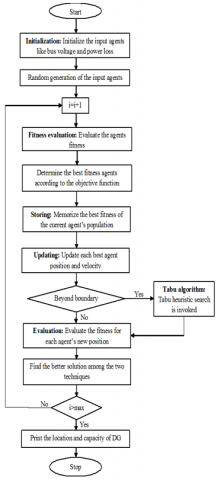Figure 1. structure of the proposed hybrid technique

## f2.png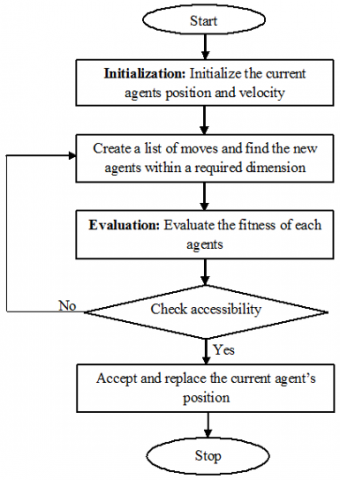Figure 2. Structure of the Tabu search algorithm

4. Results and Discussions

The established mutual technique is performed at MATLAB / simulink 7.10.0 (R2012a) platform, 4 GB of RAM, and Intel (R) core (TM) i5. Here, DG investment cost minimization, loss operating cost and DG maintenance cost is examined as intention function. The established method gets optimal location as well as sizes of DG depend on objective function, which improved planning of DG in distribution system. IEEE standard reference system, like 33 bus radial distribution, employed to plan DG at distribution system. The efficiency of established hybrid technique is recognized by comparative evaluation to GSA method. Structure of IEEE 33 radial distribution system mentioned [1, 36] at next Figure 3. Parameters of proposed technique is depicted in Table 1.

Table 1. Parameters of proposed technique

 Parameters Values Number of agents 100 Initial value of gravitational constant 100 Maximum number of iteration 100

## f3.png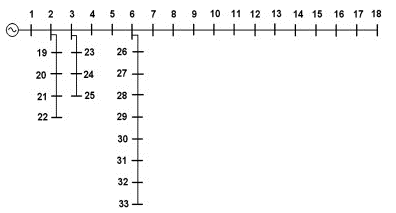Figure 3. IEEE 33 bus distribution system structure

## f4.png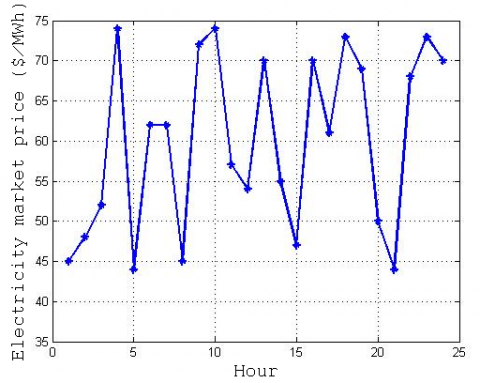Figure 4. Variable electricity market price of hourly curve

Figure 4 explains variable electricity market price of hourly curve for the distribution. From the Figure, we obtained that the 24 hours electricity market price. The IEEE 33 bus radial distribution system has 33 nodes. Voltage profile of all the nodes during the whole planning period is explained in the Figure 5. Here, the 5 years of distribution system planning voltage profile with the base year has been explained. It was seen that the voltage profile in base case maintains the normal voltage limit, which has been violated during the change of load demand in every year. The bus voltage profile variation due to the load change mentioned at next Figures.

## f5.png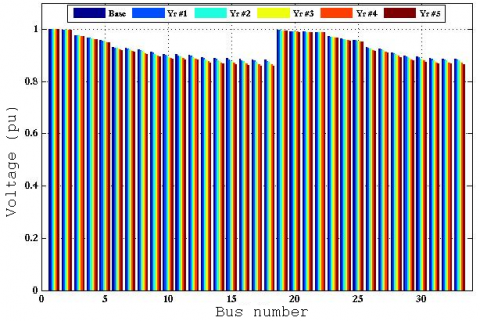Figure 5. Distribution system voltage profile at each year

## f6.png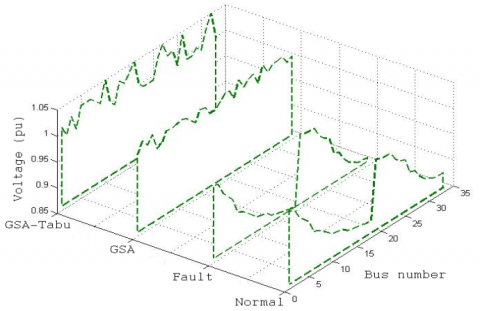Figure 6. Bus voltage profile at planning year 1

## f7.png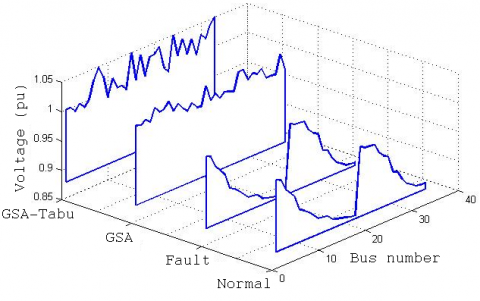Figure 7. Bus voltage profile at planning year 2

Bus voltage profile of IEEE 33 radial distribution system in the planning period of the first year is shown in the following Figure 6. Similarly the other 4 year of distribution system planning is explained in the Figures 7, 8, 9 and 10. Here bus voltage profile for normal condition, during the fault condition, GSA is the proposed hybrid technique. In the year of planning the load change as 5% to 25 % of total system load demand. It was seen that the normal condition of the system bus voltages are maintained at the secured limit, which is varied at the fault time (load increasing period). In this critical situation the bus voltage limits are adjusted by the optimum selection of DG with optimum capacitance. DG planning using GSA is not sure to minimize the voltage profile deviation of IEEE 33 bus distribution system. But proposed method solves the improper voltage deviation of the distribution system. The line losses of IEEE 33 bus radial distribution system at each year planning period is given in the following Figures 11, 12, 13, 14 and 15. From the Figures, efficiency of established technique is experienced by using IEEE 33 bus radial distribution line losses to normal condition, due to failure condition, GSA and established hybrid method. Year of planning have the load changes from 5% to 25 % of total system load demand. It was seen that in normal condition the system line losses are maintained at the secured limit. The normal line losses are increased due to the variation to the variation of the load at each year. This critical situation the line losses are minimized by the optimum selection of the DG with optimum capacity at minimum cost. The DG planning using GSA is not sure to minimize the line losses of IEEE 33 bus distribution system. But proposed method solves the line losses of the distribution system.

## f8.png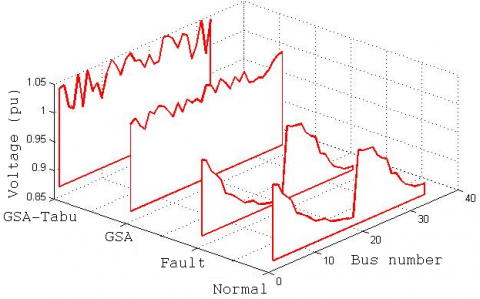Figure 8. Bus voltage profile at planning year 3

## f9.png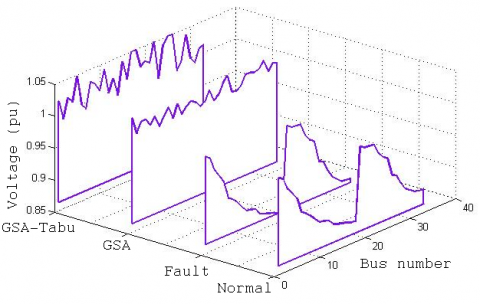Figure 9. Bus voltage profile at planning year 4

## f10.png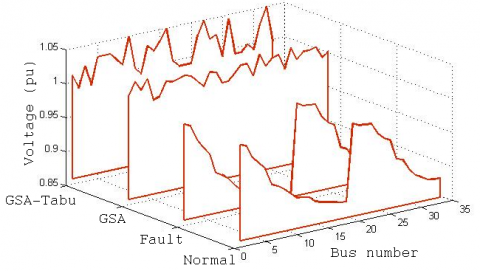Figure 10. Bus voltage profile at planning year 5

## f11.png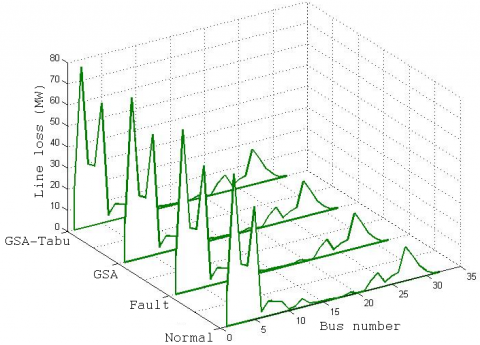Figure 11. Line losses at planning year 1

## f12.png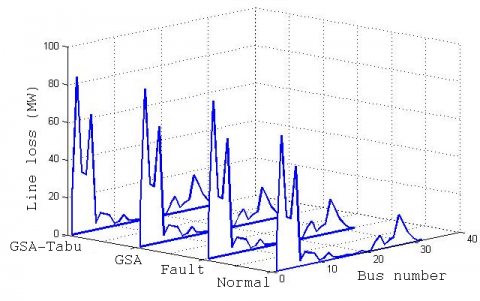Figure 12. Line losses at planning year 2

## f13.png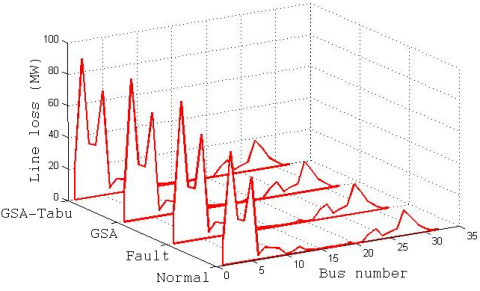Figure 13. Line losses at planning year 3

## f14.png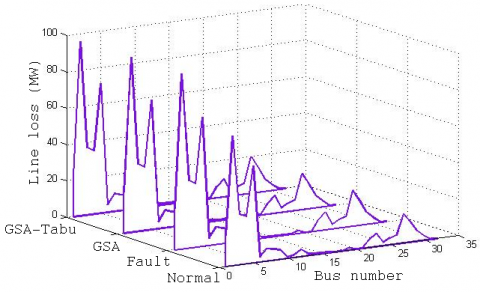Figure 14. Line losses at planning year 4

## f15.png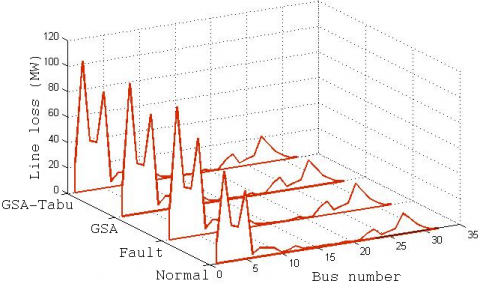Figure 15. Line losses at planning year 5

## f16.png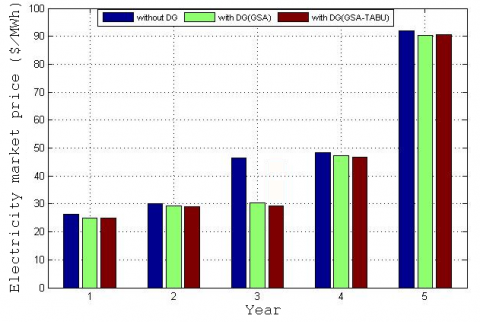Figure 16. Electricity market price after DG installation in every year

## f17.pngFigure 17. Optimum location and DG size to different techniques

The Figure 16 describes the electricity market price subsequent to DG installation in every year. Here the electricity market price has been analyzed for 5 years with different techniques such as IEEE 33 radial distribution system without DG, using GSA optimizing technique and proposed hybrid technique. In the whole period of analysis it is explained about the effective planning of DG at distribution system. During planning of DG increasing number of load demand at each year is considered. The electricity market price at whole planning period without DG have high amount of cost, the average being 49.2%. By using the GSA technique the electricity market price is slightly reduced at 44.4%. The market electricity price is 42.8% by using the proposed hybrid method at 5 years.

Optimal location and DG size employing various methods explained at Figure 18. Here, voltage profile is evaluated employing various methods such as normal state of IEEE 33 radial distribution system, employing GSA optimization technique and proposed hybrid technique. As Figure, the voltage profile of radial distribution system at normal conditions has a stable condition. Then, the voltage profile of distribution system is violated due to load demand at 5 years. In the situation, the efficiency of established technique is compared to GSA technique. It was seen that from the Figure, established technique efficiently manages voltage profile of distribution system.

## f18.png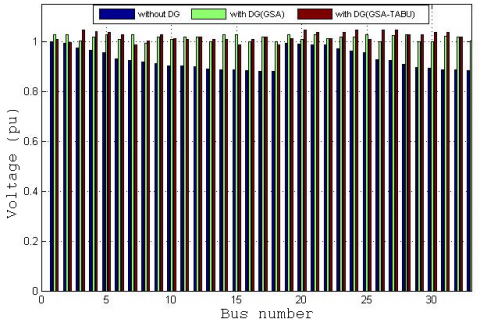Figure 18. Optimum location and DG size for different techniques

## f19.png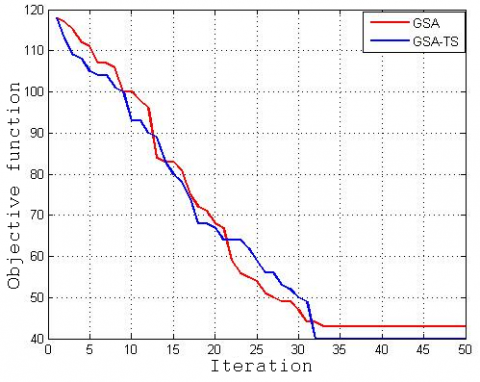Figure 19. Comparison of convergence characteristic of objective function

## f20.png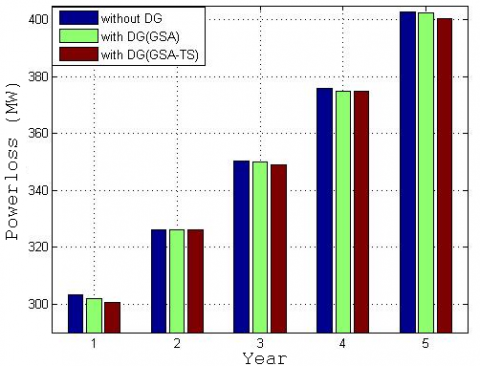Figure 20. Comparison of power loss using different techniques

Figure 19 explains the convergence feature of multi-objective function comparison that requires 50 iteration numbers to entire methods. It displays that multi-objective evaluation function uses established hybrid method as well as GSA technique. By clearly observing the Figure, GSA creates great values due to repetitions that begins as 120 and ends at 45. Because, it minimized the Distribution Generator investment cost, distribution cost system losses, operation and maintenance cost at inefficient manner. But the proposed hybrid technique takes accelerates the convergence characteristics with the combination of Tabu heuristic search, it demonstrates multi-objective function efficiently, i.e., first repetition condition of objective function denotes 120; slowly decreased due to variant number of repetitions as well as terminates 50 repetition as objective function denotes 40.

Then the power loss of radial distribution system of IEEE 33 bus employing various methods such as without DG, GSA as well as established method is explained at Figure 20 for a planning period of 5 years. In the whole period of analysis explained on the effective DG plan at distribution system. During the planning of the DG, bus power loss is considered, because of load demand at each year. The power losses at whole planning period without DG have high losses, which has 10% high from the GSA technique. The GSA contains 5% high losses compare to the proposed hybrid technique. The efficiency of established technique is verified via Table 2. IEEE 33 bus distribution system planning during whole planning period using GSA as well as established technique is illustrated. Here, established technique efficiently selects better position as well as DG capacity with low cost.

Table 2. Optimum solutions for the different methods

 Year Load (%) Best location Best capacity (MW) Total expansion cost(\$/MWh) GSA Proposed GSA Proposed GSA Proposed 1 5 2 28 2 28 1.0011 1.0011 1.0003 18.2304 18.2287 18.2287 2 10 5 2 5 2 1.0002 1.0000 23.8403 23.8380 3 15 27 5 27 5 1.0024 1.0013 23.1700 23.1677 4 20 1 4 1 4 1.0033 1.0011 28.0808 28.0800 28.0809 5 25 27 6 27 6 1.0020 1.0004 35.5495 35.5486
5. Conclusion

This manuscript established a multi-objective hybrid algorithm to plan the electrical distribution system. Here, electrical distribution system planning is done by the combined GSA and tabu heuristic search method. The GSA actively minimized the DG investment cost, cost of losses, operation as well as DG maintenance cost, which is used to optimally locate the DG with optimum capacity. Gravitational search algorithm (GSA) accelerates the speed of convergence to combination of Tabu heuristic search method search scheme. The proposed method is experienced at IEEE 33 radial bus distribution system as well as performance was evaluated to various techniques like mentioned distribution system without DG as well as GSA. The outcomes of the comparison were taken for loss of power of radial distribution system, voltage in several conditions and cost. From the comparison results we finalized as established technique as most effectual method for planning electrical distribution system that is capable in excess of other methods.

References

 Thang, V., Khanh, B., Thong, D. (2013). A new model applied to the distribution system planning for competitive electricity markets. International Journal of Energy Engineering, 3(6): 239-248. https://doi.org/10.5963/ijee0306005

 Ganguly, S., Sahoo, N., Das, D. (2011). Mono- and multi-objective planning of electrical distribution networks using particle swarm optimization. Applied Soft Computing, 11(2): 2391-2405. https://doi.org/10.1016/j.asoc.2010.09.002

 Arya, R., Choube, S., Arya, L. (2012). Reliability evaluation and enhancement of distribution systems in the presence of distributed generation based on standby mode. International Journal of Electrical Power & Energy Systems, 43(1): 607-616. https://doi.org/10.1016/j.ijepes.2012.05.045

 Singh, S., Ghose, T., Goswami, S. (2012). Optimal feeder routing based on the bacterial foraging technique. IEEE Transactions on Power Delivery, 27(1): 70-78. https://doi.org/10.1109/tpwrd.2011.2166567

 Bhowmik, S., Goswami, S., Bhattacherjee, P. (2000). A new power distribution system planning through reliability evaluation technique. Electric Power Systems Research, 54(3): 169-179. https://doi.org/10.1016/s0378-7796(99)00086-3

 Ganguly, S., Sahoo, N., Das, D. (2013). Multi-objective particle swarm optimization based on fuzzy-Pareto-dominance for possibilistic planning of electrical distribution systems incorporating distributed generation. Fuzzy Sets and Systems, 213: 47-73. https://doi.org/10.1016/j.fss.2012.07.005

 Ouyang, W., Cheng, H., Zhang, X., Yao, L. (2010). Distribution network planning method considering distributed generation for peak cutting. Energy Conversion and Management, 51(12): 2394-2401. https://doi.org/10.1016/j.enconman.2010.05.003

 Lotero, R., Contreras, J. (2011). Distribution system planning with reliability. IEEE Transactions on Power Delivery, 26(4): 2552-2562. https://doi.org/10.1109/tpwrd.2011.2167990

 Lavorato, M., Rider, M., Garcia, A., Romero, R. (2010). A constructive heuristic algorithm for distribution system planning. IEEE Transactions on Power Systems, 25(3): 1734-1742. https://doi.org/10.1109/tpwrs.2009.2038164

 Humayd, B.A., Bhattacharya, K. (2013). Comprehensive multi-year distribution system planning using back-propagation approach. IET Generation, Transmission & Distribution, 7(12): 1415-1425. https://doi.org/10.1049/iet-gtd.2012.0706

 Porkar, S., Poure, P., Abbaspour-Tehrani-fard, A., Saadate, S. (2010). A novel optimal distribution system planning framework implementing distributed generation in a deregulated electricity market. Electric Power Systems Research, 80(7): 828-837. https://doi.org/10.1016/j.epsr.2009.12.008

 Porkar, S., Poure, P., Abbaspour-Tehrani-fard, A., Saadate, S. (2010). A novel optimal distribution system planning framework implementing distributed generation in a deregulated electricity market. Electric Power Systems Research, 80(7): 828-837. https://doi.org/10.1016/j.epsr.2009.12.008

 Cory, B. (2001). Editorial: Electric utility deregulation and restructuring. IEE Proceedings - Generation, Transmission and Distribution, 148(2): 177. https://doi.org/10.1049/ip-gtd:20010328

 Zou, K., Agalgaonkar, A., Muttaqi, K., Perera, S. (2012). Distribution system planning with incorporating DG reactive capability and system uncertainties. IEEE Transactions on Sustainable Energy, 3(1): 112-123. https://doi.org/10.1109/tste.2011.2166281

 Ganguly, S., Sahoo, N., Das, D. (2010). A novel multi-objective PSO for electrical distribution system planning incorporating distributed generation. Energy Systems, 1(3): 291-337. https://doi.org/10.1007/s12667-010-0014-5

 Jahromi, M.E., Ehsan, M., Meyabadi, A.F. (2012). A dynamic fuzzy interactive approach for DG expansion planning. International Journal of Electrical Power & Energy Systems, 43(1): 1094-1105. https://doi.org/10.1016/j.ijepes.2012.06.017

 Santos, H., Legey, L. (2013). A model for long-term electricity expansion planning with endogenous environmental costs. International Journal of Electrical Power & Energy Systems, 51: 98-105. https://doi.org/10.1016/j.ijepes.2013.02.018

 Hemmati, R., Hooshmand, R., Khodabakhshian, A. (2013). State-of-the-art of transmission expansion planning: Comprehensive review. Renewable and Sustainable Energy Reviews, 23: 312-319. https://doi.org/10.1016/j.rser.2013.03.015

 Sahoo, N., Ganguly, S., Das, D. (2011). Simple heuristics-based selection of guides for multi-objective PSO with an application to electrical distribution system planning. Engineering Applications of Artificial Intelligence, 24(4): 567-585. https://doi.org/10.1016/j.engappai.2011.02.007

 Reyes-Sierra, M., Coello, C.C.A. (2006). Multi-objective particle swarm optimizers: A survey of the state-of-the-art. International Journal of Computational Intelligence Research, 2(3). https://doi.org/10.5019/j.ijcir.2006.68

 Transpire Online. (2020). Gravitational Search Algorithm (GSA) in view of Newton’s Law of Gravitation. https://transpireonline.blog/2019/07/23/gravitational-search-algorithm-gsa-in-view-of-newtons-law-of-gravitation/, accessed on 13 June 2020.

 Mythili, S., Thiyagarajah, K., Rajesh, P., Shajin, F.H. (2020). Ideal position and size selection of Unified Power Flow Controllers (UPFCs) to upgrade the dynamic stability of systems: An antlion optimiser and invasive weed optimisation algorithm. HKIE Transactions, 27: 25-37. https://doi.org/10.33430/V27N1THIE-2018-0024

 Kashem, M., Jasmon, G., Ganapathy, V. (2000). A new approach of distribution system reconfiguration for loss minimization. International Journal of Electrical Power & Energy Systems, 22(4): 269-276. https://doi.org/10.1016/s0142-0615(99)00057-5

 Rueda, J., Guaman, W., Cepeda, J., Erlich, I., Vargas, A. (2013). Hybrid approach for power system operational planning with smart grid and small-signal stability enhancement considerations. IEEE Transactions on Smart Grid, 4(1): 530-539. https://doi.org/10.1109/tsg.2012.2222678

 Ganguly, S., Sahoo, N., Das, D. (2013). Multi-objective planning of electrical distribution systems using dynamic programming. International Journal of Electrical Power & Energy Systems, 46: 65-78. https://doi.org/10.1016/j.ijepes.2012.10.030

 Naderi, E., Seifi, H., Sepasian, M. (2012). A dynamic approach for distribution system planning considering distributed generation. IEEE Transactions on Power Delivery, 27(3): 1313-1322. https://doi.org/10.1109/tpwrd.2012.2194744

 Samui, A., Samantaray, S., Panda, G. (2012). Distribution system planning considering reliable feeder routing. IET Generation, Transmission & Distribution, 6(6): 503. https://doi.org/10.1049/iet-gtd.2011.0682

 Cossi, A., da Silva, L., Lázaro, R., Mantovani, J. (2012). Primary power distribution systems planning taking into account reliability, operation and expansion costs. IET Generation, Transmission & Distribution, 6(3): 274. https://doi.org/10.1049/iet-gtd.2010.0666

 da Rocha, M., Saraiva, J. (2013). A discrete evolutionary PSO based approach to the multiyear transmission expansion planning problem considering demand uncertainties. International Journal of Electrical Power & Energy Systems, 45(1): 427-442. https://doi.org/10.1016/j.ijepes.2012.09.009

 Gitizadeh, M., Vahed, A., Aghaei, J. (2013). Multistage distribution system expansion planning considering distributed generation using hybrid evolutionary algorithms. Applied Energy, 101: 655-666. https://doi.org/10.1016/j.apenergy.2012.07.010

 Duque, F.G., De Oliveira, L.W., De Oliveira, E.J., Dias, B.H., Moraes, C.A. (2020). Modified monkey search technique applied for planning of electrical energy distribution systems. In Frontier Applications of Nature Inspired Computation, 240-265. https://doi.org/10.1007/978-981-15-2133-111

 Xie, S., Hu, Z., Yang, L., Wang, J. (2020). Expansion planning of active distribution system considering multiple active network managements and the optimal load-shedding direction. International Journal of Electrical Power & Energy Systems, 115: 105451. https://doi.org/10.1016/j.ijepes.2019.105451

 Gantayet, A., Mohanty, S., Samantaray, A.A., Satapathy, L.M., Patra, A.K., Mishra, A.K. (2020). Optimal distribution network planning with maximal benefits of loss reduction and voltage stability development. In Advances in Electrical Control and Signal Systems, 1-12. https://doi.org/10.1007/978-981-15-5262-51

 Soroudi, A., Ehsan, M., Zareipour, H. (2011). A practical eco-environmental distribution network planning model including fuel cells and non-renewable distributed energy resources. Renewable Energy, 36(1): 179-188. https://doi.org/10.1016/j.renene.2010.06.019

 Liu, S., Mernik, M., Hrnčič, D., Črepinšek, M. (2013). A parameter control method of evolutionary algorithms using exploration and exploitation measures with a practical application for fitting Sovova's mass transfer model. Applied Soft Computing, 13(9): 3792-3805. https://doi.org/10.1016/j.asoc.2013.05.010

 Porkar, S., Poure, P., Abbaspour-Tehrani-fard, A., Saadate, S. (2010). A novel optimal distribution system planning framework implementing distributed generation in a deregulated electricity market. Electric Power Systems Research, 80(7): 828-837. https://doi.org/10.1016/j.epsr.2009.12.008